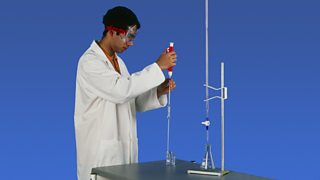# Volumetric titrationsStudents use titration apparatus to measure volumes

A titration experiment can be carried out to accurately measure the volume of substances that react in chemical reactions.

This technique utilises a standard solution (a solution of an accurately known concentration) which is titrated against portions of an unknown concentration until the reaction is just complete.

This end-point can be shown by using an indicator. Volumetric titrations are popular in acid-base reactions.

When titrating, it is essential to measure things as accurately as possible.

The experiment is carried out by following these steps:

### Step one

If the sample is a solid, it is weighed using an accurate balance, and then dissolved to make up a known volume of solution (usually 100 cm3).

### Step two

A pipette is used to measure accurately a volume of this solution - for example, 10 cm3. A safety pipette filler is used to draw solution into the pipette. This is emptied into a conical flask.

### Step three

A few drops of an indicator may be added to the conical flask. This will show a change of colour when the titration is complete.

### Step four

A second chemical is placed in a burette. This other solution is of a chemical that will react with the synthesised chemical sample in the conical flask. Often the solution in the burette is an acid of a precise, known concentration.

### Step five

The solution from the burette is run into the conical flask. The solution is added one drop at a time, with swirling to mix the solutions as the end-point is approached. Eventually, a colour change shows that the correct amount has been added to react completely with the synthesised chemical in the sample.

### Step six

The volume of solution added from the burette is noted. The titration is repeated until concordant results (within 0·2 cm3 of each other) are obtained. The titration results can then be used to calculate the amount of the synthesised chemical in the sample, and therefore find its purity.